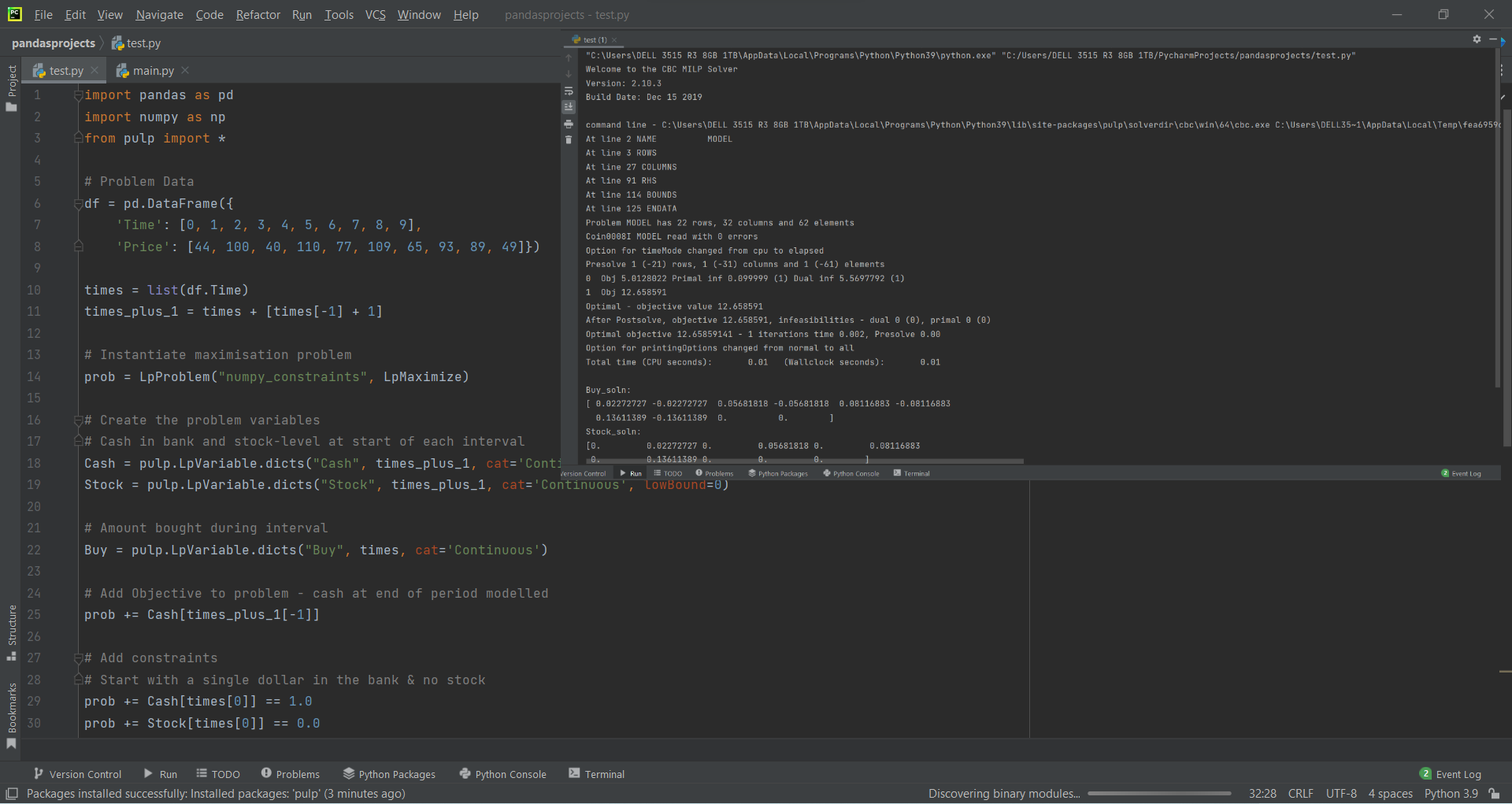# How to Maximize Revenue using Pandas in Pythonby Abdul Rawoof A RUpdated: Mar 24, 2023Solution Kit

In Python, the money that is produced by carrying out normal business operations and is calculated by multiplying the average sales price of the product by the number of items sold is called Revenue, and it is the total sum of money from which other costs and expenses are subtracted to calculated net income.

You need to give a lot of details. Still, I assume you consider the "perfect foresight" revenue maximization problem. I.e., we know at the start how the price will evolve over the horizon, and this problem is quite easy to solve, but as far as we can tell, our problem needs to be solved. We could make an arbitrarily large revenue by buying an infinite Number of units at a low price and selling them at a high price. We need to add a constraint that you can only start with a finite amount of cash and that we can only sell stock we own (this is not strictly true. It is possible to "short-sell," where you sell stuff now on the expectation its value will fall (when you will have to buy it again later). Ignoring short-selling shenanigans, we can formulate an optimization problem as a Linear Program.

Libraries that we are using to find the maximum Revenue in Python:

• Pandas: It is used to work with data sets and has functions for analyzing, exploring, manipulating data, and cleaning.
• NumPy: It is used to work with arrays and has functions for working in the domain of linear algebra and matrices.
• PuLP: It can be used to solve linear programming problems, and it is also used to solve optimization problems and has uses in various industries like Food, Transportation, etc.

Here is an example of how to maximize Revenue using Pandas in Python:Fig : Preview of the output that you will get on running this code from your IDE.

### Code

In this solution we're using Pandas, NumPy and PuLP libraries.

### Instructions

Follow the steps carefully to get the output easily.

2. Copy the snippet using the 'copy' and paste it in your IDE.
3. Add required dependencies and import them in Python file.
4. Run the file to generate the output.

I hope you found this useful. I have added the link to dependent libraries, version information in the following sections.

I found this code snippet by searching for 'how to maximize revenue using pandas' in kandi. You can try any such use case!

### Environment Tested

I tested this solution in the following versions. Be mindful of changes when working with other versions.

1. The solution is created in PyCharm 2021.3.
2. The solution is tested on Python 3.9.7.
3. Pandas version-v1.5.2.
4. NumPy version-v1.24.0.
5. PuPL version-2.7.0.

Using this solution, we are able to maximize revenue using pandas in python with simple steps. This process also facilities an easy way to use, hassle-free method to create a hands-on working version of code which would help us to maximize revenue using pandas in python.

### Dependent Libraries

pandasby pandas-dev

Python38499Version:v2.0.2Flexible and powerful data analysis / manipulation library for Python, providing labeled data structures similar to R data.frame objects, statistical functions, and much more

Support
Quality
Security
Reuse

pandasby pandas-dev

Python38499Version:v2.0.2License: Permissive (BSD-3-Clause)

Flexible and powerful data analysis / manipulation library for Python, providing labeled data structures similar to R data.frame objects, statistical functions, and much more
Support
Quality
Security
Reuse

numpyby numpy

Python23587Version:v1.24.3The fundamental package for scientific computing with Python.

Support
Quality
Security
Reuse

numpyby numpy

Python23587Version:v1.24.3License: Permissive (BSD-3-Clause)

The fundamental package for scientific computing with Python.
Support
Quality
Security
Reuse

pulpby coin-or

Python1683Version:2.7.0A python Linear Programming API

Support
Quality
Security
Reuse

pulpby coin-or

Python1683Version:2.7.0License: Others (Non-SPDX)

A python Linear Programming API
Support
Quality
Security